You are here: Start » AVL.NET » Path Features

# Path Features

Icon Name Description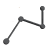PathArrayPoints Converts an array of paths to an array of points.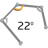PathAverageTurnAngle Computes the average absolute turn angle of a path per unit of length.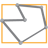PathBoundingBox Computes the smallest box containing a path.PathBoundingBox_OrNil Computes the smallest box containing a path; returns NIL if the path is empty.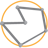PathBoundingCircle Computes the smallest circle enclosing a path.PathBoundingCircle_OrNil Computes the smallest circle enclosing a path; returns NIL if the path is empty.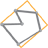PathBoundingParallelogram Computes the smallest parallelogram containing a path.PathBoundingRectangle Computes the smallest rectangle containing a path.PathBoundingRectangle_FixedAngle Computes the smallest rectangle with the given orientation angle containing a path.PathBoundingRectangle_FixedAngle_OrNil Computes the smallest rectangle with the given orientation angle containing a path; returns NIL when the path is empty.PathBoundingRectangle_OrNil Computes the smallest rectangle containing a path; returns NIL when the path is empty.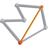PathCaliperDiameter Computes the longest and the shortest width of the input path measured as distance between parallel lines containing the whole path.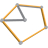PathConvexHull Computes the smallest convex shape that contains the given path.PathDiameter Finds the longest segment connecting two characteristic points of a path.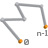PathEndpoints Returns the two endpoints of a path.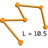PathLength Computes the total length of the input path.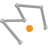PathMassCenter Computes the average of the path points (all, not only characteristic ones).PathMassCenter_OrNil Computes the average of the path points (all, not only characteristic ones); returns NIL if the path is empty.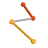PathSegments Converts a path to an array of line segments.PathSelfIntersections Checks if a path has any self-intersections and computes all of them.PathSize Returns the number of characteristic points on the input path.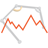PathTurnAngleLocalMaxima Detection of feature points related to object corners.PathTurnAngleMaximum Finds the maximum of the profile of turn angles of a path.PathTurnAngleMaximum_OrNil Finds the maximum of the profile of turn angles of a path; returns NIL if the path has no turn angles.PathTurnAngleProfile Computes the profile of turn angles at characteristic points of a path.